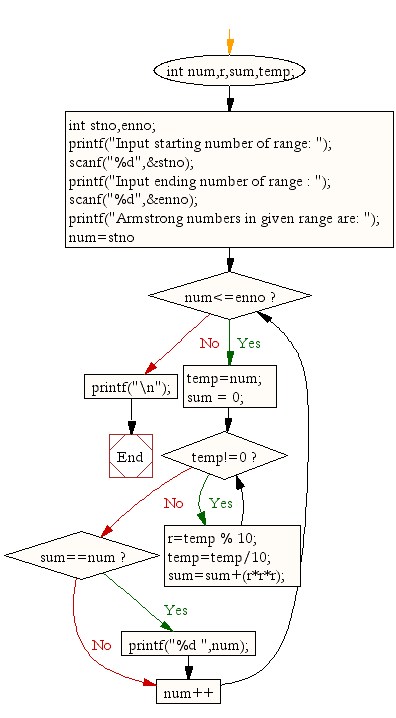﻿ C : Find the Armstrong number for a given range of number# C Exercises: Find the Armstrong number for a given range of number

## C For Loop: Exercise-30 with Solution

Write a C program to find the Armstrong number for a given range of number.

Sample Solution:

C Code:

``````/*When the sum of the cube of the individual digits of a number
is equal to that number, the number is called Armstrong number. For example 153.
Sum of its divisor is 13 + 53;+ 33; = 1+125+27 = 153*/
#include <stdio.h>

void main(){
int num,r,sum,temp;
int stno,enno;

printf("Input starting number of range: ");
scanf("%d",&stno);

printf("Input ending number of range : ");
scanf("%d",&enno);

printf("Armstrong numbers in given range are: ");
for(num=stno;num<=enno;num++){
temp=num;
sum = 0;

while(temp!=0){
r=temp % 10;
temp=temp/10;
sum=sum+(r*r*r);
}
if(sum==num)
printf("%d ",num);
}
printf("\n");
}
```
```

Sample Output:

```Input starting number of range: 1
Input ending number of range : 1000
Armstrong numbers in given range are: 1 153 370 371 407
```

Flowchart:C Programming Code Editor:

Improve this sample solution and post your code through Disqus.

What is the difficulty level of this exercise?

Test your Programming skills with w3resource's quiz.

﻿

## C Programming: Tips of the Day

How to initialize array to 0 in C?

Global variables and static variables are automatically initialized to zero. If you have simply-

`char ZEROARRAY;`

at global scope it will be all zeros at runtime. But actually there is a shorthand syntax if you had a local array. If an array is partially initialized, elements that are not initialized receive the value 0 of the appropriate type. You could write:

`char ZEROARRAY = {0};`

The compiler would fill the unwritten entries with zeros. Alternatively you could use memset to initialize the array at program startup:

Ref : https://bit.ly/3cvqplP